# Samacheer Kalvi 9th Maths Solutions Chapter 3 Algebra Ex 3.7

## Tamilnadu Samacheer Kalvi 9th Maths Solutions Chapter 3 Algebra Ex 3.7

9th Maths Exercise 3.7 Question 1.
Find the quotient and remainder of the following.
(i) (4x3 + 6x2 – 23x + 18) ÷ (x + 3)
(ii) (8y3 – 16y2 + 16y -15) ÷ (2y – 1)
(iii) (8x3 – 1) ÷ (2x – 1)
(iv) (-18z + 14z2 + 24z3 + 18) ÷ (3z + 4)
Solution:
(i) (4x3 + 6x2 – 23x + 18) ÷ (x + 3)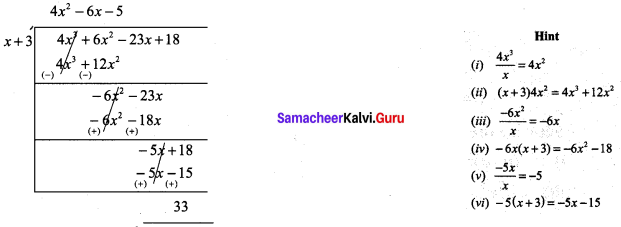Quotient = 4x2 – 6x – 5
Remainder = 33

(ii) (8y3 – 16y2 + 16y -15) ÷ (2y – 1)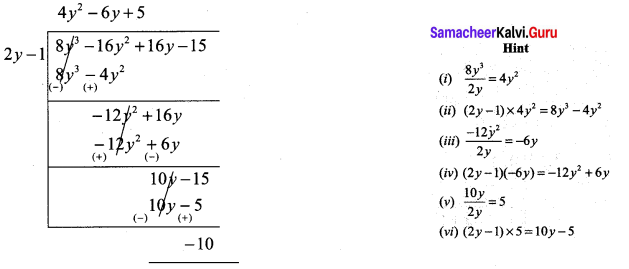Quotient = 4y2 – 6y + 5
Remainder = -10

(iii) (8x3 – 1) ÷ (2x – 1)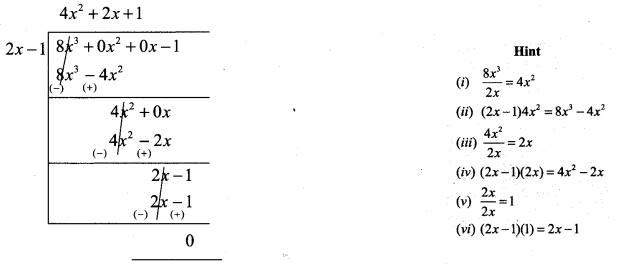Quotient = 4x2 + 2x + 1
Remainder = 0

(iv) (-18z + 14z2 + 24z3 + 18) ÷ (3z + 4)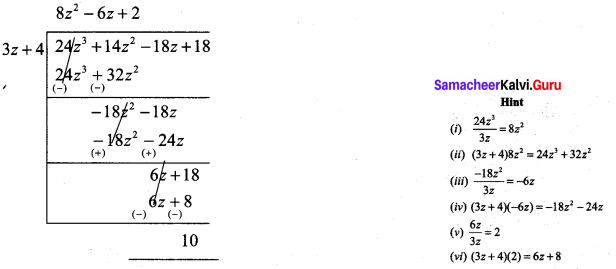Quotient = 8z2 – 6z + 2
Remainder = 10

9th Maths Algebra Exercise 3.7 Question 2.
The area of rectangle is x2 + 7x + 12. If its breadth is (x + 3), then find its length.
Solution:
Area of a rectangle = x2 + 7x + 12
Its breadth = x + 3
x2 + 7x + 12 = (x + 3) × length
∴ Length = (x2 + 7x + 12) ÷ (x + 3)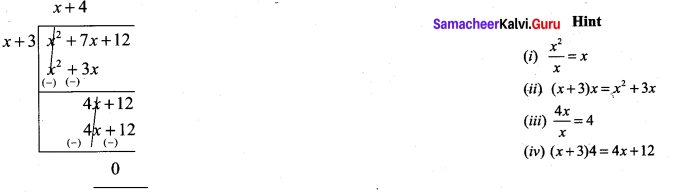Quotient = x + 4; Remainder = 0;
∴ Length = x + 4

Samacheer Kalvi 9th Maths Question 3.
The base of a parallelogram is (5x + 4). Find its height, if the area is 25x2 – 16.
Solution:
The base of a parallelogram is (5x + 4)
Area = 25x2 – 16
Area = b × h = 25x2 – 16
base = 5x + 4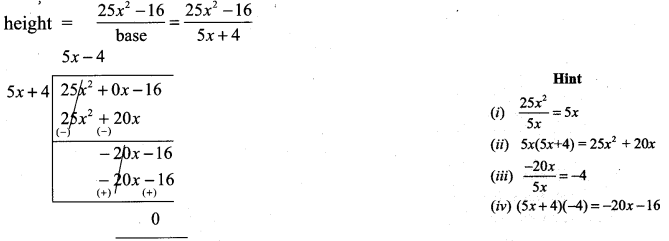∴ height of the parallelogram = 5x – 4.

9th Maths Samacheer Kalvi Question 4.
The sum of (x + 5) observations is (x3 + 125). Find the mean of the observations.
Solution:
The sum of (x + 5) observations is (x3 + 125)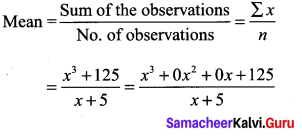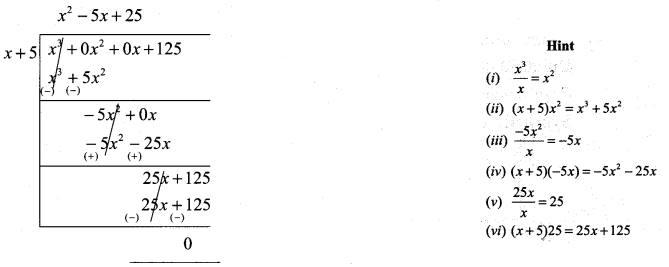Quotient = x2 – 5x + 25
Remainder = 0
∴ Mean of the observations = x2 – 5x + 25Samacheerkalvi.Guru 9th Maths Question 5.
Find the quotient and remainder for the following using synthetic division:
(i) (x3 + x2 – 7x – 3) ÷ (x – 3)
(ii) (x3 + 2x2 – x – 4) ÷ (x + 2)
(iii) (3x3 – 2x2 + 7x – 5) ÷ (x + 3)
(iv) (8x4 – 2x2 + 6x + 5) ÷ (4x + 1)
Solution:
(i) (x3 + x2 – 7x – 3) ÷ (x – 3)
Let p (x) = x3 + x2 – 7x – 3
q (x) = x – 3 To find the zero of x – 3
p(x) in standard form ((i.e.) descending order)
x3 + x2 – 7x – 3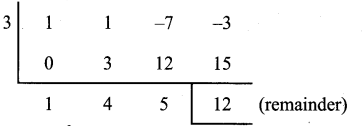Quotient is x2 + 4x + 5
Remainder is 12

(ii) (x3 + 2x2 – x – 4) + (x + 2)
p (x) = x3 + 2x2 – x – 4
Co-efficients are 1 2 -1 – 4
To find zero of x + 2, put x + 2 = 0; x = -2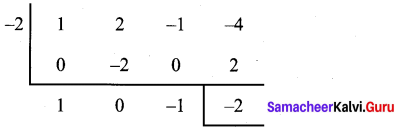∴ Quotient is x2 – 1
Remainder is -2

(iii) (3x3 – 2x2 + 7x – 5) ÷ (x + 3)
To find zero of the divisor (x + 3), put x + 3 = 0; ∴ x = – 3
Dividend in Standard form 3x3 – 2x2 + 7x – 5
Co-efficients are 3 -2 7 – 5
Synthetic Division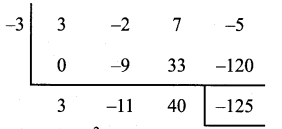Quotient is 3x2 – 11x + 40
Remainder is -125

(iv) (8x4 – 2x2 + 6x + 5) ÷ (4x + 1)
To find zero of the divisor 4x + 1, put 4x + 1 = 0 ; 4x = -1; x = $$-\frac{1}{4}$$
Dividend in Standard form 8x4 + 0x3 – 2x2 + 6x + 5
Co-efficients are 8 0 -2 6 5
Synthetic Division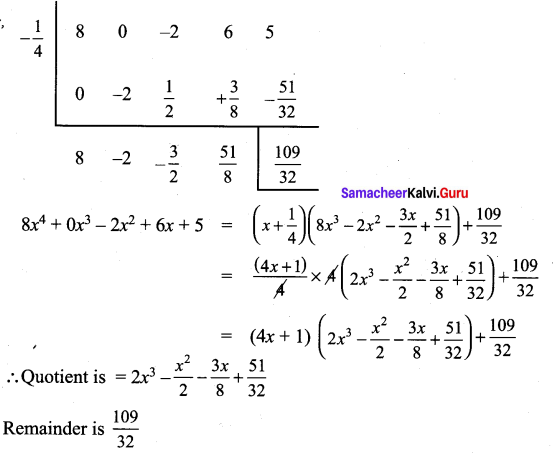Samacheer Kalvi Maths 9th Question 6.
If the quotient obtained on dividing (8x4 – 2x2 + 6x – 7) by (2x + 1) is (4x3 + px2 -qx + 3), then find p, q and also the remainder.
Solution:
Let p (x) = 8x4 – 2x2 + 6x – 7
Standard form = 8x4 + 0x3 – 2x2 + 6x – 7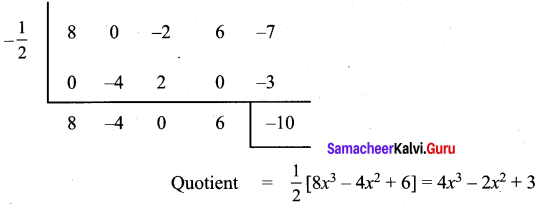Quotient 4x3 – 2x2 + 3 is compared with the given quotient 4x3 + px2 – qx + 3
Co-efficients of x2 is p = – 2
Co-efficients of x is q = 0
Remainder is – 10

9th Maths Algebra Question 7.
If the quotient obtained on dividing 3x3 + 11x2 + 34x + 106 by x – 3 is 3x2 + ax + b, then find a, b and also the remainder.
Solution:
Let p (x) = 3x3 + 11x2 + 34x + 106
p (x) in standard form
Co-efficients are 3 11 34 106
q(x) = x – 3, its zero x = 3
Synthetic division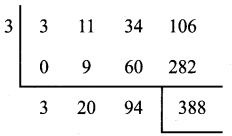Quotient is 3x2 + 20x + 94, it is compared with the given quotient 3x2 + ax + b
Co-efficient of x is a = 20
Constant term is b = 94
Remainder r = 388